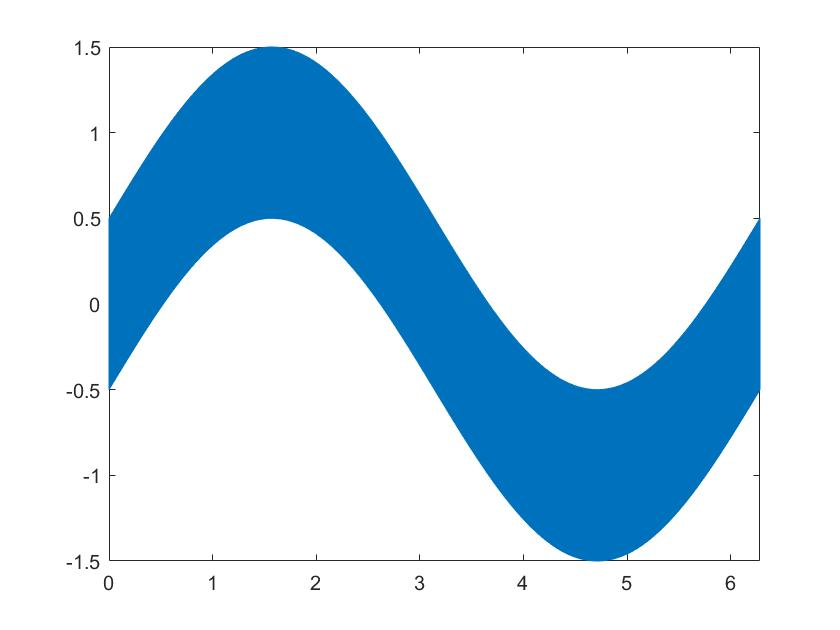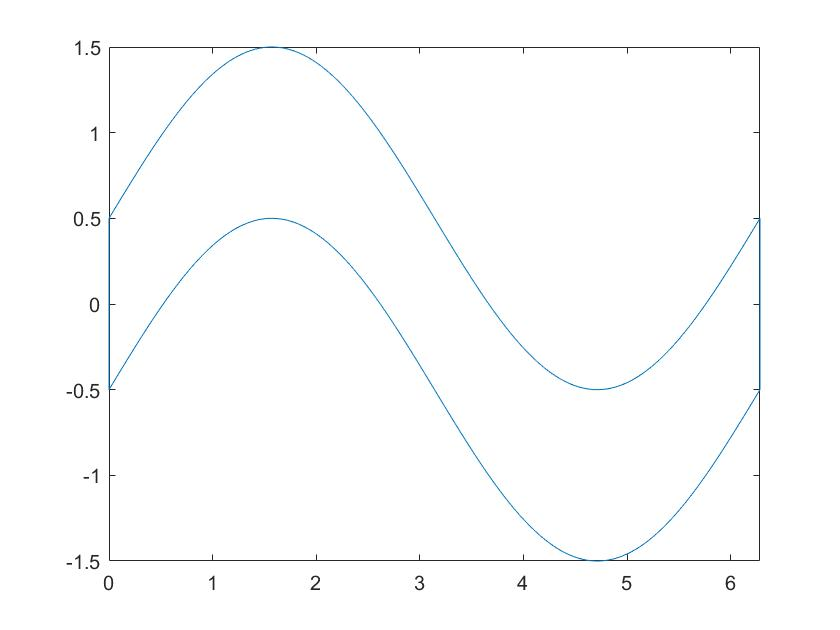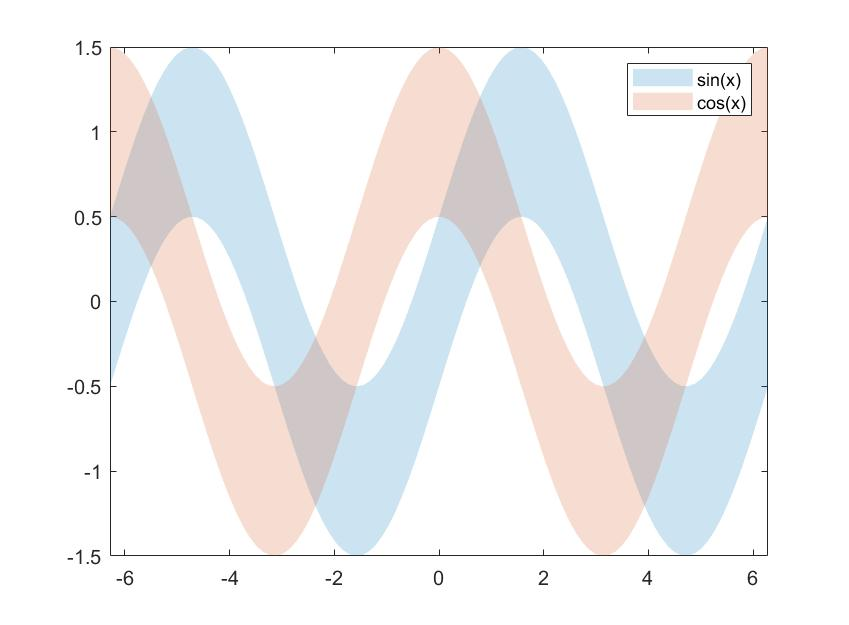## Plotting Uncertainty (Bounded Line)

version 1.0.4 (3.47 KB) by
Display the upper and lower uncertainty bounds about X and Y datasets in a highly customizable style

Updated Wed, 17 Aug 2022 18:02:17 +0000

Description
Plot the 2-D uncertainty bounds (upper and lower) about a standard 2-D line plot of x and y data. The bounds are assumed to be +/- of the specified uncertainty value about the y data. This routine features a variable number of user input properties allowing the user to specify customized settings for both the built-in plot and patch routines.
Syntax
plotUnc(x,y,u,l)
plotUnc(x,y,u,l,ColorSpec)
plotUnc(y,u,l)
plotUnc(y,u,l,ColorSpec)
plotUnc(__,Name,Value)
plotUnc(ax, __)
h = plotUnc(__)
Examples
Create an Uncertainty Plot
Create x as a vector of linearly spaced values between 0 and 2π. Use an increment of π/100 between the values. Create y values equal to the sine of x and display a bounded region of ±0.5 about y as defined by the upper and lower bounds (ub and lb).
x = 0:pi/100:2*pi;
y = sin(x);
ub = ones(size(x))./2;
lb = ones(size(x))./2;
plotUnc(x,y,ub,lb);Plot Uncertainty Without Shading
In some instances, it may be desirable to display bounds about a 2-D dataset without shading. Using the previous example, this can be customized by adding input arguments for the patch routine
plotUnc(x,y,ub,lb,'FaceColor','none');Plot Multiple Uncertainties
Create x as a vector of 100 linearly spaced values between -2π and 2π. The underlying y data sets are defined as sine and cosine values of x. Plot the uncertainties about both data sets and set their transparency.
x = linspace(-2*pi,2*pi,100)';
y1 = sin(x);
y2 = cos(x);
ub = ones(size(y1))./2;
lb = ones(size(y1))./2;
plotUnc([x,x],[y1,y2],[ub,ub],[lb,lb],'EdgeColor','none','FaceAlpha',0.2);
legend('sin(x)','cos(x)');### Cite As

Darin Koblick (2022). Plotting Uncertainty (Bounded Line) (https://www.mathworks.com/matlabcentral/fileexchange/116385-plotting-uncertainty-bounded-line), MATLAB Central File Exchange. Retrieved .

##### MATLAB Release Compatibility
Created with R2021a
Compatible with any release
##### Platform Compatibility
Windows macOS Linux

### Community Treasure Hunt

Find the treasures in MATLAB Central and discover how the community can help you!

Start Hunting!